﻿ Reliability Level of Al-Mn Alloy Corrosion Rate Dependence on Its As-Cast Manganese Content and Pre-Installed Weight in Sea Water Environment

### Reliability Level of Al-Mn Alloy Corrosion Rate Dependence on Its As-Cast Manganese Content and Pre-...

C. I. Nwoye, P. C. Nwosu, E. C. Chinwuko, S. O. Nwakpa, I. E. Nwosu, N. E. IdenyiOPEN ACCESSPEER-REVIEWED

## Reliability Level of Al-Mn Alloy Corrosion Rate Dependence on Its As-Cast Manganese Content and Pre-Installed Weight in Sea Water Environment

C. I. Nwoye1,, P. C. Nwosu2, E. C. Chinwuko3, S. O. Nwakpa1, I. E. Nwosu4, N. E. Idenyi5

1Department of Metallurgical and Materials Engineering, Nnamdi Azikiwe University, Awka, Nigeria

2Department of Mechanical Engineering, Federal Polytechnic Nekede, Nigeria

3Department of Industrial and Production Engineering, Nnamdi Azikiwe University, Awka, Nigeria

4Department of Environmental Technology, Federal University of Technology, Owerri, Nigeria

5Department of Industrial Physics Ebonyi State University, Abakiliki, Nigeria

### Abstract

The corrosion rate of Al-Mn alloy in sea water environment was studied, considering the catastrophic effect of salty water on aluminum alloy. The reliability level of the alloy corrosion rate dependence on its as-cast manganese content and pre-installed weight under service condition; in sea water environment was evaluation. Analysis of the surface structures of corroded Al-Mn alloys were carried out to evaluate the phase distribution morphology. The reliability response coefficient of the alloy corrosion rate to the combined influence of as-cast manganese content ϑ and pre-installed alloy weight ɤ were evaluated to ascertain the reliability of the highlighted dependence. Analysis of the surface structure of the corroded alloy revealed in all cases widely distributed oxide film of the alloy in whitish form. Increased oxidation of the as-cast Al-Mn matix (up to 3%Mn input) was due to increased permeability of the formed oxide film (to corrosive species in the sea water) as result of increased as-cast manganese content of the alloy (up to 3% Mn). Above 3% Mn input, the corrosion rate dropped indicating oxide film coherency and resistance to inflow of oxygen into the alloy. A two-factorial polynomial model was derived, validated and used for the predictive evaluation of the Al-Mn alloy corrosion rate. The validity of the model;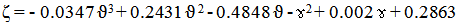was rooted on the core model expression ζ + ɤ2 - 0.002 ɤ - 0.2863 = - 0.0347 ϑ3 + 0.2431 ϑ 2 - 0.4848 ϑ where both sides of the expression are correspondingly approximately equal. The corrosion rate per unit as-cast manganese content as obtained from experiment and derived model were 0.0714 and 0.0714 mm/yr/ % respectively. Standard errors incurred in predicting the corrosion rate for each value of the as-cast manganese content & pre-installed alloy weight considered as obtained from experiment and derived model were 0.0333 and 0.03346 % & 0.0350 and 0.0363 % respectively. Deviational analysis indicates that the maximum deviation of model-predicted corrosion rate from the experimental results was less than 9%. This translated into over 91% operational confidence and response level for the derived model as well as over 0.91 reliability response coefficient of corrosion rate to the collective operational contributions of as-cast manganese content and pre-installed alloy weight in the sea environment.

### At a glance: Figures

1234
Prev Next

• Nwoye, C. I., et al. "Reliability Level of Al-Mn Alloy Corrosion Rate Dependence on Its As-Cast Manganese Content and Pre-Installed Weight in Sea Water Environment." American Journal of Mining and Metallurgy 2.3 (2014): 57-63.
• Nwoye, C. I. , Nwosu, P. C. , Chinwuko, E. C. , Nwakpa, S. O. , Nwosu, I. E. , & Idenyi, N. E. (2014). Reliability Level of Al-Mn Alloy Corrosion Rate Dependence on Its As-Cast Manganese Content and Pre-Installed Weight in Sea Water Environment. American Journal of Mining and Metallurgy, 2(3), 57-63.
• Nwoye, C. I., P. C. Nwosu, E. C. Chinwuko, S. O. Nwakpa, I. E. Nwosu, and N. E. Idenyi. "Reliability Level of Al-Mn Alloy Corrosion Rate Dependence on Its As-Cast Manganese Content and Pre-Installed Weight in Sea Water Environment." American Journal of Mining and Metallurgy 2, no. 3 (2014): 57-63.

 Import into BibTeX Import into EndNote Import into RefMan Import into RefWorks

### 1. Introduction

The susceptibility of aluminum and its alloys to corrosion attack and its attendant abrupt in-service failures have raised an urgent need for intensive and extensive research & development geared towards controlling and inhibiting the corrosion attack. Achievement of a high level of quality and safety assurance in the selection and usage of metals and alloys requires a comprehensive and intensive study of the corrosion type, its mode of attack, its testing, evaluation as well as monitoring.

Metallic corrosion has been reported  to be basically electrochemical in nature, involving both oxidation and reduction reactions. During oxidation, the resulting metal ions may either go into the corroding solution or form an insoluble compound since there is loss of the metal atom’s valence electrons. The researcher concluded that these electrons are transferred to at least one other chemical species during reduction. The scientist further reveal that the character of the corrosion environment dictates which of several possible reduction reactions will occur.

A number of metals and alloys passivate, or lose their chemical reactivity significantly, under some environmental circumstances called to play [2, 3]. This phenomenon basically involve the formation of a thin protective oxide film which varies with wet-dry cycle, atmospheric conditions, type and amount of pollutants, the chemical composition and metallurgical history of the metals or alloys as well as physico-chemical properties of coating. Research  carried out on stainless steels and aluminum alloys revealed that they both exhibit this type of behavior. Results of the research showed that the alloy’s S-shaped electrochemical potential-versus-log current density curve ideally explains the phenomenon: active-to-passive behavior. The research also showed that intersections with reduction polarization curves in active and passive regions correspond, respectively, to high and low corrosion rates.

The high level of resistance of aluminum and its alloys to corrosion in many environments stems significantly on their ability to passivates. The protective films rapidly reforms if damaged. However, it was reported  that a change in the character of the environment (e.g., alteration in the concentration of the active corrosive species) may cause a passivated material to revert to an active state. The report revealed that a sharp increase in corrosion rate, by as much as 100,000 times could result from subsequent damage to a preexisting passive film. The behavior is linear as it is for normal metals at relatively low potential values, within the “active” region. Furthermore, the current density suddenly decreases to a very low value that remains independent of potential with increasing potential. This is referred to as the “passive” region. Finally, the current density again increases with potential in the “transpassive” region at even higher potential values.

Al-Mn alloys has been reported  to be susceptible to corrosion attack if exposed in the atmosphere because of the presence of moisture. The corrosion of this alloy stems from the strong affinity aluminium has for oxygen which results to its oxidation and subsequent formation of oxide film. Similar research  revealed that with time, this film becomes passive to further oxidation and stable in aqueous media when the pH is between 4.0 and 8.5. It is important to state that the passive films can break and fall of, hence exposing the surface of the alloy to further corrosion.

Studies [1, 6, 7, 8] have shown series of methods for calculating the corrosion rate. Even though there is an electric current associated with electrochemical corrosion reactions, corrosion rate can be expressed in terms of this current, or, more specifically, current density- that is, the current per unit surface area of material corroding, which is designated i . The corrosion rate r, in units of mol/m2s, is therefore determined using the expression;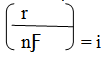(1)

where, n is the number of electrons associated with the ionization of each metal atom, and is 96,500 C/mol.

The possible exposure time for aluminium-manganese alloy (having particular corrosion rates and as-cast weights) in sea water environment has been evaluated  using a validated empirical model. The validity of the derived model;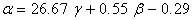(2)

was rooted on the core expression: 0.0375 α = γ + 0.0206 β – 0.0109 where both sides of the expression are correspondingly approximately equal. The depth of corrosion penetration (at increasing corrosion rate: 0.0104-0.0157 mm/yr) as predicted by derived model and obtained from experiment are 0.7208 x 10-4 & 1.0123 x 10-4 mm and 2.5460 x 10-4 & 1.8240 x 10-4 mm (at decreasing corrosion rate: 0.0157-0.0062 mm/yr) respectively. Statistical analysis of model-predicted and experimentally evaluated exposure time for each value of as-cast weight and alloy corrosion rate considered shows a standard error of 0.0017 & 0.0044 % and 0.0140 & 0.0150 % respectively. Deviational analysis indicates that the maximum deviation of the model-predicted alloy exposure time from the corresponding experimental value is less than 10%.

Assessment of the open system corrosion rate of aluminium-manganese alloy in sea water environment was carried out  based on the alloy weight loss and exposure time. A model was derived and used as a tool for the assessment. It is made up of a quadratic and natural logarithmic function. The validity of the model;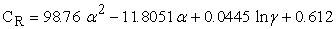(3)

was rooted on the core expression: 1.0126 x10-2 CR = α2 – 11.9538 x 10-2 α + 4.5059 x10-4 lnγ + 6.1968 x 10-3 where both sides of the expression are correspondingly approximately equal. The resultant depth of corrosion penetration as predicted by derived model, regression model and obtained from experiment are 0.0102, 0.01 and 0.0112 mm respectively, while the corrosion rate per unit weight loss of the alloy as predicted by derived model, regression model and obtained from experiment are 7.7830, 7.6774 and 8.5777 mm/yr/g respectively. The maximum deviation of the model-predicted alloy corrosion rates from the corresponding experimental values is less than 27%. Statistical analysis of model-predicted, regression-predicted and experimentally evaluated corrosion rates for each value of exposure time and alloy weight loss considered shows a standard error of 0.0657, 0.0709 & 0.0715 % and 0.0190 & 2.83 x 10-5 & 0.0068 % respectively.

The predictability of Al-Mn alloy corrosion rate in atmospheric environment was assessed  based on direct input of initial weights of the alloy and its exposure times. The validity of the two-factorial polynomial derived model;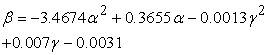(4)

is rooted on the core expression 0.2884 β = - α2 + 0.1054α – 3.7489 x 10-4 γ2 + 2.0186 x 10-3γ – 8.9396 x 10-4 where both sides of the expression are correspondingly approximately equal. Corrosion rate per unit initial weight of exposed alloy as predicted by derived model and obtained from experiment are 1.8421 and 1.6316 (mm/yr) kg-1 respectively. Similarly, between exposure time: 0.0192 - 0.0628 yr, the depth of corrosion penetration on the exposed alloy as predicted by derived model and obtained from experiment are 1.5260 x 10-4 and 1.3516 x 10-4 mm respectively. Deviational analysis indicates that the maximum deviation of the model-predicted corrosion penetration rate from the corresponding experimental value is less than 11%.

Statistical analysis of model-predicted and experimentally evaluated corrosion rates as well as depth of corrosion penetration for each value of alloy initial weight and exposure time considered show standard errors of 0.0014 and 0.0015 % as well as 9.48 x10-4 and 8.64 x10-4 %, respectively.

The aim of this research is to ascertain the reliability level of Al-Mn alloy corrosion rate dependence on its as-cast manganese content and its pre-installed weight in sea water environment. The difference between the present and past researches  and  is just that the possible exposure time of Al-Mn in the sea water environment was evaluated based on the alloy as-cast weight and its corrosion rate  while in , the corrosion rate of the same alloy was predicted based on its exposure time and weight loss in the sea water environment.

### 2. Materials and Methods

Materials used for the experiment are virgin aluminium of 99% purity and pure granulated manganese. The other materials used were acetone, sodium chloride, distilled water, beakers and measuring cylinders. The equipment used were lathe machine, drilling machine, crucible furnace, analytical digital weighing machine, reagents for etching and metallurgical microscope.

2.1. Specimen Preparation and Experimentation

Computation for each of the Al-Mn alloy compositions was carefully worked out, and the alloying materials charged into the surface crucible furnace. The molten alloy was cast into cylindrical shapes and allowed to cool in air (at room temperature). The cooled cylinders were machined to specific dimensions, cut into test samples and weighed. Each sample coupon was drilled with 5mm drill bit to provide hole for the suspension of the strings and submersion of the sample in sea water environment.

The surface of each of the test coupons was thoroughly polished (before and after corrosion) with emery cloth according to ASTM standards.

The method adopted for this phase of the research is the weight loss technique. The test coupons were exposed to the sea water environment and withdrawn after a known period of time. The withdrawn coupons were washed with distilled water, cleaned with acetone and dried in open air before weighing to determine the final weight.

### 3. Results and Discussion

3.1. Surface Structural Analysis of Corroded Al-Mn Alloy
Figure 3. Surface structure of Al-Mn alloy (a) control (b), (c) (d), (e) and (f) for as-cast manganese contents: 2, 2.5, 2.8, 3 and 4 wt % respectively. (x200)

Figure 3(a) depicts a slightly corroded Al-Mn alloy which serves as control alloy, since it was not exposed to the sea water environment. The surface structure of this alloy shows an ash coloured background; a little away from the normal colour of aluminium, due to absence of manganese. Figure 3(a) also shows inconspicuous presence of oxide film in the control alloy exposed to room atmosphere.

Figure 3(b - f) show different levels of intensive corrosion attacks at different as-cast manganese contents. The white patches observed in Figure 3(b – f) are strongly believed to be oxide films produced during the initial corrosion attack on the Al-Mn alloy. Also, the greenish patches also observed in Figure 3(b – f) were attributed to increased oxidation of the alloy matrix as a result of increased as-cast manganese content in alloy exposed to the sea water environment.

Table 1 and Table 2 show that the corrosion rate of Al-Mn alloy increases with increase in the alloy as-cast manganese content (up to 3%). Increased alloy as-cast manganese content ensures prolonged physico-chemical interactions between the Al-Mn alloy and corrosion-induced agents resident in the sea water. Increased pre-installed weight (2-3% Mn input) increased the alloy corrosion rate through greater metal/alloy removal per unit area following activities of the corrosion-induced aggressive species (resident in the sea water) on the alloy. Table 1 and Table 2 present similar results except the conversion of alloy pre-installed weight from gramme (g) to kilogramme (Kg).

#### Table 2. Variation of corrosion rate ζ of Al-Mn alloy with its as-cast manganese content ϑ and pre-installed weight ɤ (in kg)

Aluminum and its alloys are highly corrosion resistant in many environments due to its ability to passivate, and the protection offered by the initially formed film. Table 2 suggests that increased oxidation of the as-cast Al-Mn matix (up to 3%Mn input) was due to increased permeability of the formed oxide film (to corrosive species in the sea water) as result of increased as-cast manganese content of the alloy (up to 3% Mn). Above 3% Mn input, the corrosion rate dropped (Table 2), indicating oxide film coherency and resistance to inflow of oxygen into the alloy. This implied that the increased corrosion attack (as input Mn increased up to 3%) on the alloy occurred because the passivated material initially formed on the alloy were increasingly permeable to the active corrosive species in the sea water within this as-cast Mn content.

3.1.1. Boundary and Initial Conditions

Consider solid Al-Mn alloy exposed to atmosphere environment and interacting with some corrosion-induced agents. The atmosphere is assumed to be affected by unwanted dissolved gases. Range of input manganese concentration considered: 1.0 – 4.0%. Alloy pre-installed weight range considered: 0.0118-0.0125 kg. The quantity and purity of aluminium used were 99 wt % and 99% respectively.

The boundary conditions are: aerobic environment to enhance Al-Mn alloy oxidation (since the atmosphere contains oxygen. At the bottom of the exposed alloy, a zero gradient for the gas scalar are assumed. The exposed alloy is stationary. The sides of the solid are taken to be symmetries.

Computational analysis of generated experimental data shown in Table 2, gave rise to Table 3 which indicate that;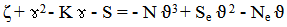(5)

Introducing the values of K, S, N, Se, and Ne into equation (5) reduces it to;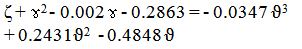(6)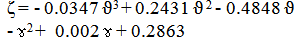(7)

Where K = 0.002, S = 0.2863, N = 0.0347, Se= 0.2431 and Ne = 0.4848 are empirical constants (determined using C-NIKBRAN 

(ϑ) = As-cast manganese content (%)

(ɤ) =Alloy pre-installed weight (kg)

(ζ) = Corrosion rate (mm/yr)

The derived model is equation (7). Computational analysis of Table 2 gave rise to Table 3. The derived model is two-factorial polynomial in nature since it is composed of two input process factors: as-cast manganese content and alloy pre-installed weight. This implies that the predicted corrosion rate of Al-Mn alloy in the sea water environment is dependent on just two factors: as-cast manganese content and alloy pre-installed weight.

#### Table 3. Variation of ζ + ɤ2 - 0.002 ɤ - 0.2863 with - 0.0347 ϑ3 + 0.2431ϑ 2 - 0.4848 ϑ

3.2. Model Validity

The validity of the model is strongly rooted on equation (6) (core model equation) where both sides of the equation are correspondingly approximately equal. Table 3 also agrees with equation (6) following the values of ζ + ɤ2 - 0.002 ɤ - 0.2863 and - 0.0347 ϑ3 + 0.2431 ϑ 2 - 0.4848 ϑ evaluated from the experimental results in Table 1. Furthermore, the derived model was validated by comparing the corrosion rate predicted by the model and that obtained from the experiment. This was done using various analytical techniques.

3.2.1. Statistical Analysis

3.2.1.1. Standard Error (STEYX)

The standard errors incurred in predicting Al-Mn corrosion rate for each value of as-cast manganese content & pre-installed weight considered as obtained from experiment and derived model were 0.0333 and 0.03346 % & 0.0350 and 0.0363 % respectively. The standard error was evaluated using Microsoft Excel version 2003.

3.2.1.2. Correlation

The correlation coefficient between Al-Mn corrosion rate and as-cast manganese content and alloy pre-installed weight were evaluated (using Microsoft Excel Version 2003) from results of the experiment and derived model. These evaluations were based on the coefficients of determination R2 shown in Figure 4- Figure 7.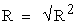(8)
Figure 4. Coefficient of determination between Al-Mn alloy corrosion rate and as-cast manganese content as obtained from the experiment
Figure 5. Coefficient of determination between Al-alloy Mn corrosion rate and as-cast manganese content as predicted by derived model
Figure 6. Coefficient of determination between Al-Mn alloy corrosion rate and its pre-installed weight as obtained from the experiment
Figure 7. Coefficient of determination between Al-Mn alloy corrosion rate and its pre-installed weight as obtained from the experiment

#### Table 4. Comparison of the correlations between corrosion rate and as-cast manganese content as evaluated from experimental (ExD) and derived model (MoD) predicted results

The evaluated correlations are shown in Tables 4 and 5. These evaluated results indicate that the derived model predictions are significantly reliable and hence valid considering its proximate agreement with results from actual experiment.

#### Table 5. Comparison of the correlations between corrosion rate and pre-installed weight and as evaluated from experimental and derived model predicted results

3.3. Graphical Analysis

Graphical analysis of Figure 8 and Figure 9 shows very close alignment of the curves from derived model and experiment. It is strongly believed that the degree of alignment of these curves is indicative of the proximate agreement between ExD and MoD predicted results.

Figure 8. Comparison of the Al-Mn alloy corrosion rate (relative to as-cast manganese content) as obtained from experiment and derived model
Figure 9. Comparison of the Al-Mn corrosion rate (relative to alloy pre-installed weight) as obtained from experiment and derived model
3.4. Computational Analysis

Computational analysis of the experimental and model-predicted corrosion rate per unit as-cast manganese content was carried out to ascertain the degree of validity of the derived model. This was done by comparing the corrosion rate per unit as-cast manganese content obtained by calculation, using experimental and model-predicted results.

Corrosion rate of Al-Mn alloy per unit as-cast manganese content during the period of exposure in sea water environment ζS (mm/yr /%) was calculated from the equation;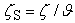(9)

Rewritten as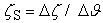(10)

Equation (10) is detailed as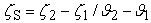(11)

Where

Δζ = Change in the corrosion rates (ζ2 - ζ1) at two values of input manganese concentration ϑ2 , ϑ 1

Δϑ = Change in the pre-installed weights ϑ 2, ϑ 1

Considering the points (2, 0.0115) & (3, 0.0829), and (2, 0.0114) & (3, 0.0828) as shown in Figure 4, 5 and 8 then designating them as (ζ1, ϑ1) & (ζ2, ϑ2) for experimental and derived model predicted results respectively, and also substituting them into equation (11), gives positive slopes: 0.0714 and 0.0714 mm/yr /% as their corrosion rate per unit input manganese concentration respectively.

3.5 Deviational Analysis

Comparative analysis of the corrosion rates precisely obtained from experiment and derived model shows that the model-predicted values deviated from experimental results. This was attributed to the fact that the effects of the surface properties of the Al-Mn alloy which played vital roles during the corrosion process were not considered during the model formulation. This necessitated the introduction of correction factor, to bring the model-predicted corrosion rate to those of the corresponding experimental values.

The deviation Dv, of model-predicted corrosion rate from the corresponding experimental result was given by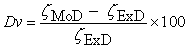(12)

Where ζExD and ζMoD are corrosion rates evaluated from experiment and derived model respectively.

Figure 10. Variation of model-predicted corrosion rate with associated deviation from experimental results (relative to as-cast manganese content)

Figure 10 and Figure 11 show that the least and highest magnitudes of deviation of the model-predicted corrosion rate (from the corresponding experimental values) are – 0.12 and + 8.89%.

Figure 11. Variation of model-predicted corrosion rate with associated deviation from experimental results (relative to pre-installed weight)

It could be seen from Figure 10 and Figure 11 that these deviations correspond to corrosion rates: 0.0828 and 0.0514 mm/yr, as-cast manganese contents: 3 and 2.5% as well as alloy pre-installed weights: 0.0121 and 0.0124 kg respectively.

Comparative analysis of Figure 10 and Figure 11 also shows that the maximum deviation of model-predicted corrosion rate from the experimental results was less than 9%. This translated into over 91% operational confidence and response level for the derived model as well as over 0.91 reliability response coefficient of corrosion rate to the collective operational contributions of the as-cast manganese content and pre-installed alloy weight (under service) in the sea water environment.

Correction factor, Cf to the model-predicted results was given by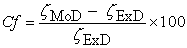(13)

Analysis of Table 6 in comparison with Figure 10 and 11 indicates that the evaluated correction factors are negative of the deviation as shown in equations (12) and (13).

The correction factor took care of the negligence of operational contributions of the effects of surface properties of the Al-Mn alloy which actually affected the corrosion process. The model predicted results deviated from those of the experiment because these contributions were not considered during the model formulation. Introduction of the corresponding values of Cf from equation (13) into the model gives exactly the corresponding experimental corrosion rate

#### Table 6. Variation of correction factor with as-cast manganese content and pre-installed alloy weight

Table 6 shows that the least and highest magnitudes of correction factor to the model-predicted corrosion rate are + 0.12 and - 8.89%. Since correction factor is the negative of deviation (equations (12) and (13)), Table 6, Figure 10 and 11 indicate that these correction factors correspond to corrosion rates: 0.0828 and 0.0514 mm/yr, as-cast manganese contents: 3 and 2.5% as well as alloy pre-installed weights: 0.0121 and 0.0124 kg respectively.

It is important to state that the deviation of model predicted results from that of the experiment is just the magnitude of the value. The associated sign preceding the value signifies that the deviation is a deficit (negative sign) or surplus (positive sign).

### 4. Conclusions

The reliability level of Al-Mn alloy corrosion rate dependence on its as-cast manganese content and pre-installed weight under service condition; in sea water environment has been evaluation. Increased oxidation of the as-cast Al-Mn matix (up to 3%Mn input) was due to increased permeability of the formed oxide film (to corrosive species in the sea water) as result of increased as-cast manganese content of the alloy (up to 3% Mn). Above 3% Mn input, the corrosion rate dropped indicating oxide film coherency and resistance to inflow of oxygen into the alloy. Oxide films of the corroded Al-Mn alloy were widely distributed in whitish form within the alloy surface structures. The validity of the two-factorial polynomial model derived, validated and used for the predictive evaluation. was rooted in the core model expression ζ + ɤ2 - 0.002 ɤ - 0.2863 = - 0.0347 ϑ3 + 0.2431 ϑ 2 - 0.4848 ϑ where both sides of the expression are correspondingly approximately equal. The corrosion rate per unit as-cast manganese content as obtained from experiment and derived model were 0.0714 and 0.0714 mm/yr/ % respectively. Standard errors incurred in predicting the corrosion rate for each value of the as-cast manganese content & pre-installed alloy weight considered as obtained from experiment and derived model were 0.0333 and 0.03346 % & 0.0350 and 0.0363 % respectively. Deviational analysis indicates that the maximum deviation of model-predicted corrosion rate from the experimental results was less than 9%. This translated into over 91% operational confidence and response level for the derived model as well as over 0.91 reliability response coefficient of corrosion rate to the collective operational contributions of as-cast manganese content and pre-installed alloy weight in the sea environment.

### References

  Callister Jr, W. D. (2007). Materials Science and Engineering, 7th Edition, John Wiley & Sons Inc., .In article  Ekuma, C. E., and Idenyi, N. E. (2007). Statistical Analysis of the influence of Environment on Prediction of Corrosion from its Parameters. Res. J. Phy., , 1(1):27-34.In article  Stratmann, S. G., and Strekcel, H. (1990). On the Atmospheric Corrosion of Metals which are Covered with Thin Electrolyte Layers. II. Experimental Results. Corros. Sci.., 30:697-714.In article CrossRef  Polmear, I., J. (1981). Light Alloys. Edward Arnold Publishers Ltd.In article  Ekuma, C. E., Idenyi, N. E., and Umahi, A. E. (2007). The Effects of Zinc Addition on the Corrosion Susceptibility of Aluminium Alloys in Various Tetraoxosulphate (vi) Acid Environments. J. of Appl. Sci., 7(2):237-241.In article CrossRef  Nwoye, C. I., Neife, S., Ameh, E. M., Nwobasi, A. and Idenyi, N. E. (2013). Predictability of Al-Mn Alloy Exposure Time Based on Its As-Cast Weight and Corrosion Rate in Sea Water Environment. Journal of Minerals and Materials Characterization and Engineering, 1:307-314.In article CrossRef  Nwoye, C. I., Idenyi, N. E., Asuke, A. and Ameh, E. M. (2013). Open System Assessment of Corrosion Rate of Aluminum-Manganese Alloy in Sea Water Environment Based on Exposure Time and Alloy Weight Loss. J. Mater. Environ. Sci., 4(6): 943-952. http://dx.doi.org/10.4236/jmmce.2013.16046In article  Nwoye, C. I., Idenyi, N. E., and Odo, J. U. (2012). Predictability of Corrosion Rates of Aluminum-Manganese Alloys Based on Initial Weights and Exposure Time in the Atmosphere, Nigerian Journal of Materials Science and Engineering, 3(1):8-14.In article  Nwoye, C. I. (2008). C-NIKBRAN Data Analytical Memory (Software).In article